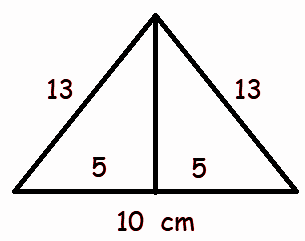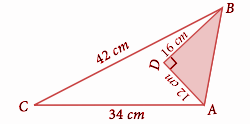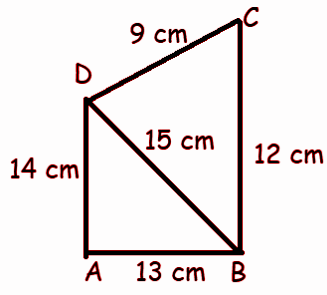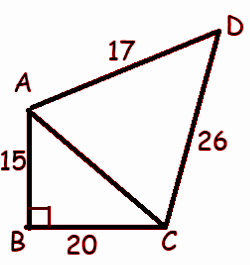# Samacheer Kalvi 9th Maths Book Solutions Chapter 7 | Mensuration Answers For Tamil Nadu Board

Samacheer Kalvi 9th Maths Book Solutions Chapter 7 – Mensuration is available here. The Samacheer Kalvi 9th Maths book answers of Chapter 7, available at BYJU’S, contain step by step explanations designed by our mathematics experts. All these important questions are based on the new pattern prescribed by the Tamil Nadu board. Students can also get the solutions of other chapters on Samacheer Kalvi 9th Maths solutions. Students can solve these Samacheer Kalvi Class 9 Maths Book Chapter 7 Questions for practice. They can also refer to the solutions given to these questions in order to gauge their performance.

## Samacheer Kalvi Class 9 Maths Book Chapter 7 Questions with Solutions

Chapter 7 of the Samacheer Kalvi 9th Maths guide will help the students to solve problems related to Heron’s formula, application of Heron’s formula in finding areas of quadrilaterals, the surface area of cuboid and cube, volume of cuboid and cube.

### Samacheer Kalvi 9th Maths Chapter 7: Trigonometry Book Exercise 7.1 Questions and Solutions

Question 1: Using Heron’s formula, find the area of a triangle whose sides are

(i) 10 cm, 24 cm, 26 cm

(ii) 1.8m, 8 m, 8.2 m

Solution:

(i) 10 cm, 24 cm, 26 cm

a = 10 cm, b = 24 cm and c = 26 cm

s = (a + b + c) / 2

s = (10 + 24 + 26) / 2

s = 60 / 2 = 30

s – a = 30 – 10 = 20

s – b = 30 – 24 = 6

s – c = 30 – 26 = 4

√s (s − a) (s − b) (s − c)

= √30 (20) (6) (4)

= 120 cm2

(ii) 1.8m, 8 m, 8.2 m

a = 1.8 m, b = 8 m and c = 8.2 m

s = (a + b + c) / 2

s = (1.8 + 8 + 8.2) / 2

s = 18 / 2 = 9

s – a = 9 – 1.8 = 7.2

s – b = 9 – 8 = 1

s – c = 9 – 8.2 = 0.8

√s(s − a)(s − b)(s − c)

= √9 (7.2) (1) (0.8)

= 7.2 m2

Question 2: The sides of the triangular ground are 22 m, 120 m and 122 m. Find the area and cost of levelling the ground at the rate of ₹ 20 per m2.

Solution:

a = 22 m, b = 120 m and c = 122 m

s = (a + b + c) / 2

s = (22 + 120 + 122) / 2

s = 264 / 2 = 132

s – a = 132 – 22 = 110

s – b = 132 – 120 = 12

s – c = 132 – 122 = 10

√s(s − a)(s − b)(s − c) = √132 (110) (12) (10)

= √(11 ⋅ 12 ⋅ 11 ⋅ 10 ⋅ 12 ⋅ 10)

= 11 ⋅ 12 ⋅ 10

= 1320 m2

Cost of levelling the ground = ₹ 20 per m2.

Required cost = 20 (1320)

= ₹ 26400

Question 3: The perimeter of a triangular plot is 600 m. If the sides are in the ratio 5:12:13, then find the area of the plot.

Solution:

Let the sides of the triangle are 5x, 12x and 13x.

The perimeter of triangular plot = 600

5x + 12x + 13x = 600

30x = 600

x = 600 / 30 = 20

5x = 5 (20) = 100

12x = 12 (20) = 240

13x = 13 (20) = 260

s = 600 / 2 = 300

s – a = 300 – 100 = 200

s – b = 300 – 240 = 60

s – c = 300 – 260 = 40

√s(s − a)(s − b)(s − c) = √300 (200) (60) (40)

= √(3 ⋅ 10 ⋅ 10 ⋅ 2 ⋅ 10 ⋅ 10 ⋅ 3 ⋅ 2 ⋅ 10 ⋅ 2 ⋅ 2 ⋅10)

= 12000 m2

Question 4: Find the area of an equilateral triangle whose perimeter is 180 cm.

Solution:

The perimeter of equilateral triangle = 180 cm

3a = 180

a = 180 / 3 = 60 cm

Area of equilateral triangle = (√3 / 4)a2

= (√3 / 4)602

= 900√3

= 900 (1.732)

= 1558.8 cm2

Question 5: An advertisement board is in the form of an isosceles triangle with perimeter 36m and each of the equal sides is 13 m. Find the cost of painting it at ₹ 17.50 per square metre.

Solution:

Let “x” be the unknown side of the triangle.

The sides of the triangle are x, 13 and 13.

perimeter = 36 m

x + 13 + 13 = 36

x + 26 = 36

x = 36 – 26 = 10 m

a = 10, b = 13 and c = 13height = √132 – 52

= √(169 – 25)

= √144

height = 12 cm

Area of triangle = (1 / 2) x base x height

= (1 / 2) x 10 x 12

= 60 cm2

Cost of painting it at ₹ 17.50 per square metre

Required cost = 60 (17.50) = ₹ 1050

Question 6: Find the area of the unshaded region.Solution:

∠BDA = 90

AB2 = DA2 + DB2

AB2 = 122 + 162

AB2 = 144 + 256

AB2 = 400

AB = 20

Area of unshaded region = Area of ACB – Area of ADB

Area of ACB :

AC = 34 cm, BC = 42 cm and AB = 20

s = (a + b + c) / 2

s = (34 + 42 + 20) / 2

s = 48

s – a = 48 – 34 = 14, s – b = 48 – 42 = 6 and s – c = 48 – 20 = 28

= √s(s – a) (s – b) (s – c)

= √48 ⋅ 14 ⋅ 6 ⋅ 28

= 336 cm2

DB = 16 cm, DA = 12 cm and AB = 20

s = (16 + 12 + 20) / 2

s = 48 / 2 = 24

s – a = 24 – 16 = 8, s – b = 24 – 12 = 12 and s – c = 24 – 20 = 4

= √24 ⋅ 8 ⋅ 12 ⋅ 4

= 96 cm2

Area of unshaded region = 336 – 96

= 240 cm2

Question 7: Find the area of a quadrilateral ABCD whose sides are AB = 13cm, BC = 12cm, CD = 9 , cm AD = 14cm and diagonal BD = 15cm.

Solution:Area of quadrilateral ABCD = Area of ADB + Area of DBC

a = 13, b = 15, c = 14

s = (13 + 15 + 14) / 2 = 21

= √21(21 – 13) (21 – 15) (21 – 14)

= √21 ⋅ 8 ⋅ 6 ⋅ 7

= 84 cm2

Area of BDC :

a = 12, b = 9, c = 15

s = (12 + 9 + 15) / 2 = 18

= √18(18 – 12) (18 – 9) (18 – 15)

= √18 ⋅ 6 ⋅ 9 ⋅ 3

= 54 cm2

Area of quadrilateral = 84 + 54

= 138 cm2

Question 8: A park is in the shape of a quadrilateral. The sides of the park are 15 m, 20 m, 26 m and 17 m and the angle between the first two sides is a right angle. Find the area of the park.

Solution:In △ABC,

AC2 = AB2 + BC2

AC2 = 152 + 202

AC2 = 225 + 400

AC = √625 = 25

Area of quadrilateral ABCD = Area of ABC + Area of ADC

Area of ABC :

a = 15, b = 20, c = 25

s = (15 + 20 + 25) / 2 = 30

= √30(30 – 15) (30 – 20) (30 – 25)

= √30 ⋅ 15 ⋅ 10 ⋅ 5

= 150 cm2

a = 17, b = 26, c = 25

s = (17 + 26 + 25) / 2 = 34

= √34(34 – 17) (34 – 26) (34 – 25)

= √34 ⋅ 17 ⋅ 8 ⋅ 9

= 204 cm2

Area of quadrilateral = 150 + 204

= 354 cm2

Question 9: A land is in the shape of a rhombus. The perimeter of the land is 160 m and one of the diagonals is 48 m. Find the area of the land.

Solution:

In a rhombus, the length of all sides is equal.

The perimeter of rhombus = 160 m

Let “x” be the side length of a rhombus.

4x = 160

x = 40 m

Area of rhombus = 2 (Area of the triangle whose sides length are 40 m, 40 m and 48 m)

s = (a + b + c) / 2

s = (40 + 40 + 48) / 2 = 64

= √s(s – a) (s – b) (s – c)

= √64 ⋅ (64 – 40) (64 – 40) (64 – 48)

= √64 ⋅ 24 ⋅ 24 ⋅ 16

= 768 m2

Area of rhombus = 2 (768) = 1536 m2

Question 10: The adjacent sides of parallelogram measure 34 m, 20 m and the measure of one of the diagonals is 42 m. Find the area of the parallelogram.

Solution:

Area of parallelogram = 2 (Area of the triangle whose sides are 34 m, 20 m and 42 m)

s = (a + b + c) / 2

s = (34 + 20 + 42) / 2 = 48

= √s(s – a) (s – b) (s – c)

= √48 ⋅ (48 – 34) (48 – 20) (48 – 42)

= √48 ⋅ 14 ⋅ 28 ⋅ 6

= 336 m2

Area of rhombus = 2 (336) = 672 m2

### Samacheer Kalvi 9th Maths Chapter 7: Trigonometry Book Exercise 7.2 Questions and Solutions

Question 1: Find the total surface area and the lateral surface area of a cuboid whose dimensions are: length = 20 cm, breadth = 15 cm and height = 8 cm.

Solution:

Total surface area is = 2(lw + wh + hl)

= 2[20(15) + 15(8) + 8(20)]

= 2 [300 + 120 + 160]

= 2(580)

= 1160 cm2

Lateral surface area is = 2h(l + w)

= 2(8)(20 + 15)

= 16(35)

= 560 cm2

Question 2: The dimensions of a cuboidal box are 6 m × 400 cm × 1.5 m. Find the cost of painting its entire outer surface at the rate of ₹22 per m2.

Solution:

Length (l) = 6 m

Width (w) = 400 cm = 400 / 100 m = 4 m

Height (h) = 1.5 m

The outer surface area has six sides.

The required area is

= 2 (lw + wh + hl)

= 2 [6(4) + 4(1.5) + 1.5(6)]

= 2 [24 + 6 + 9]

= 2 

= 78 m2

Cost of painting the surface is ₹22 per m2.

So, the required cost is

= 78(22)

= ₹1716

Question 3: The dimensions of a hall is 10 m × 9 m × 8 m. Find the cost of whitewashing the walls and ceiling at the rate of ₹8.50 per m2.

Solution:

Length (l) = 10 m

Width (w) = 9 m

Height (h) = 8 m

Area of whitewashing is = 2h (l + w) + lw

= 2 (8) (10 + 9) + 10 (9)

= 16 (19) + 90

= 304 + 90

= 394 m2

Cost of whitewashing is \$8.50 per m2.

The required cost is

= 394(8.50)

= 3349

Question 4: Find the TSA and LSA of the cube whose side is

(i) 8 m

(ii) 21 cm

(iii) 7.5 cm

Solution:

(i) 8 m

Total surface area = 6a2

Side length (a) = 8 m

= 6(8)2

= 6(64)

= 384 m2

Lateral surface area = 4a2

Side length (a) = 8 m

= 4(8)2

= 4(64)

= 256 m2

(ii) 21 cm

Total surface area = 6a2

Side length (a) = 21 cm

= 6(21)2

= 6(441)

= 2646 cm2

Lateral surface area = 4a2

Side length (a) = 21 cm

= 4(21)2

= 4(441)

= 1764cm2

(iii) 7.5 cm

Total surface area = 6a2

Side length (a) = 7.5 cm

= 6(7.5)2

= 6(56.25)

= 337.5 cm2

Lateral surface area = 4a2

Side length (a) = 7.5 cm

= 4(7.5)2

= 4(56.25)

= 225 cm2

Question 5: If the total surface area of a cube is 2400 cm2 then, find its lateral surface area.

Solution:

Total surface area = 2400

6a2 = 2400

a2 = 2400 / 6

a2 = 400

a = 20 cm

Lateral surface area = 4a2

= 4(20)2

= 4(400)

= 1600 cm2

Question 6: A cubical container of side 6.5 m is to be painted on the entire outer surface. Find the area to be painted and the total cost of painting it at the rate of ₹24 per m2.

Solution:

The side length of cubical container = 6.5 m

The curved surface area of container = 6a2

= 6(6.5)2

= 6(42.25)

= 253.5 m2

Cost of painting = ₹24 per m2

Required cost = 253.5 (24)

= ₹ 6084

Question 7: Three identical cubes of side 4 cm are joined end to end. Find the total surface area and lateral surface area of the new resulting cuboid.

Solution:

Curved surface area of resulting cuboid = 2h(l + b)

length of resulting cuboid = 4 + 4 + 4 = 12 cm

breadth of cuboid = 4 cm and height of cuboid = 4 cm

= 2 (4) (12 + 4)

= 8(16)

= 128 cm2

Total surface area 2 (lb + bh + hl)

= 2 [12(4) + 4(4) + 4(12)]

= 2 [ 48 + 16 + 48 ]

= 2 (112)

= 224 cm2

### Samacheer Kalvi 9th Maths Chapter 7: Trigonometry Book Exercise 7.3 Questions and Solutions

Question 1: Find the volume of a cuboid whose dimensions are

(i) length = 12 cm, breadth = 8 cm, height = 6 cm

(ii) length = 60 m, breadth = 25 m, height = 1.5 m

Solution:

(i) length = 12 cm, breadth = 8 cm, height = 6 cm

Volume of cuboid = l * b * h

= 12 (8) (6)

= 12 (48)

= 576 cm3

(ii) length = 60 m, breadth = 25 m, height = 1.5 m

Volume of cuboid = l * b * h

= 60 (25) (1.5)

= 60 (37.5)

= 2250 cm3

Question 2: The dimensions of a matchbox are 6 cm × 3.5 cm × 2.5 cm. Find the volume of a packet containing 12 such matchboxes.

Solution:

Length = 6 cm, breadth = 3.5 cm and height = 2.5 cm

The volume of cuboid = lbh

= 6 (3.5) (2.5)

= 52.5 cm3

Volume of 12 boxes = 52.5 (12)

= 630 cm3

Question 3: The length, breadth and height of a chocolate box are in the ratio 5:4:3. If its volume is 7500 cm3, then find its dimensions.

Solution:

Volume of box = 7500 cm3

Let 5x, 4x and 3x be the length breadth and height of chocolate boxes, respectively.

5x (4x) (3x) = 7500

60x3 = 7500

x3 = 125

x = 5 cm

length = 5(5) = 25 cm

breadth = 4(5) = 20 cm

height = 3(5) = 15 cm

Question 4: The length, breadth and depth of a pond are 20.5 m, 16 m and 8 m, respectively. Find the capacity of the pond in litres.

Solution:

Length = 20.5 m, breadth = 16 m and height = 8 m

The capacity of pond = l * b * h

= 20.5 (16) (8)

= 2624 m3

1000 liters = 1 m3

= 2624 (1000)

= 2624000 litres

Question 5: The dimensions of brick are 24 cm × 12 cm × 8 cm. How many such bricks will be required to build a wall of 20 m length, 48 cm breadth and 6 m height?

Solution:

The volume of 1 brick = l * b * h

= 24 (12) (8)

= 2304 cm3

Volume of wall = 2000 (48) (600)

= 57600000 m3

Number of bricks required = 57600000 / 2304

= 25000

The required number of bricks is 25000.

Question 6: The volume of a container is 1440 m3. The length and breadth of the container are 15 m and 8 m, respectively. Find its height.

Solution:

The volume of container = 1440 m3

length x breadth x height = 1440

15 x 8 x h = 1440

h = 1440 / 120

h = 12 m

Question 7: Find the volume of a cube each of whose side is

(i) 5 cm

(ii) 3.5 m

(iii) 21 cm

Solution:

(i) 5 cm

The volume of cube = a3 = 53 = 125

Volume of cube = 125 cm3

(ii) 3.5 m

The volume of cube = a3 = 3.53 = 42.875

Volume of cube = 42.875 cm3

(iii) 21 cm

The volume of cube = a3 = 213 = 9261

Volume of cube = 9261 cm3

Question 8: A cubical milk tank can hold 125000 litres of milk. Find the length of its side in metres.

Solution:

The volume of cubical milk tank = 125000 litres

1 m3 = 1000 liter

The volume of the tank in m3 = 125000 / 1000

a3 = 125

a = 5 m

Question 9: A metallic cube with side 15 cm is melted and formed into a cuboid. If the length and height of the cuboid is 25 cm and 9 cm, respectively then find the breadth of the cuboid.

Solution:

The volume of cube = Volume of the cuboid

a3 = 153

The volume of cube = 3375

length x breadth x height = 3375

25 x breadth x 9 = 3375

breadth = 3375 / (25 x 9)

= 15 m